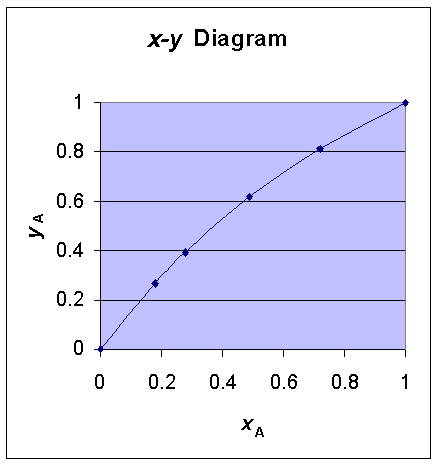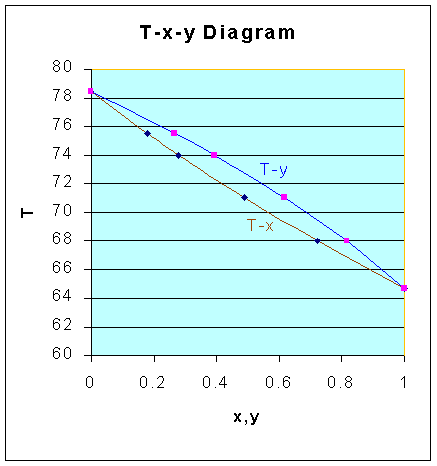### Vapor-Liquid Equilibrium Data

Methanol and ethanol form an ideal solution. Compute vapor-liquid equilibrium data and prepare plots of x-y and T-x-y at 1 atm pressure. The following pure component vapor pressure data is given:

 Vapor pressure, mm Hg 200 400 760 1520 Temperature oC for ethanol 48.4 62.5 78.4 97.5 Temperature oC for methanol 34.8 49.9 64.7 84

What value of relative volatility will you recommend for this system?

Calculations:

Boiling point for the solution will range from the pure component boiling point of low boiling component to the pure component boiling point of high boiling component.

In this case, the boiling point of solution will range from 64.7oC to 78.4oC.

According to Clasius-Clapeyron equation, for short ranges of temperatures ln P Vs 1/T (temperature in Kelvin) is a straight line.

We can utilize this linear relationship fact, for interpolation of vapor pressure for various temperatures.

For methanol and ethanol we want to have the vapor pressure data for the temperature range of 64.7 to 48.4oC.

Interpolating equation for methanol: (using the data for T of 64.7 and 84oC)

(ln 760 - ln PA) / (ln 760 - ln 1520) = (1/337.7 - 1/T) / (1/337.7 - 1/357)

Interpolating equation for ethanol: (using the data for T of 62.5 and 78.4oC)

(ln 400 - ln PB) / (ln 400 - ln 760) = (1/335.5 - 1/T) / (1/335.5 - 1/351.4)

The vapor pressure data for various pressures are calculated using the above equations and tabulated as follows:

 T, oC T, Kelvin ln PA PA ln PB PB 64.7 337.7 6.6333 760 6.0839 439 68 341 6.7574 860 6.2203 503 71 344 6.8681 961 6.3420 568 74 347 6.9769 1072 6.4616 640 75.5 348.5 7.0307 1131 6.5206 679 78.4 351.4 7.1332 1253 6.6333 760

Using these vapor pressure - temperature data, and from the relation:

xA = (Pt - PB)/(PA - PB) and yA = PAxA/Pt

and Relative volatility, a = PA/PB

x-y plot T-x-y plot data are calculated as follows:

 T, oC PA, mm Hg PB, mm Hg xA yA a 64.7 760 439 1 1 1.73 68 860 503 0.720 0.815 1.71 71 961 568 0.489 0.618 1.69 74 1072 640 0.278 0.392 1.68 75.5 1131 679 0.179 0.267 1.67 78.4 1253 760 0 0 1.65 Average a = 1.69The recommended relative volatility for the system is 1.69.